[BACK]
 Intelligent Automation & Soft ComputingDOI:10.32604/iasc.2021.019067Article

Performances of K-Means Clustering Algorithm with Different Distance Metrics

1Center for Cyber Security, Faculty of Information Science and Technology, Universiti Kebansaan Malaysia (UKM), 43600, Bangi, Selangor, Malaysia
2School of Information Technology, Skyline University College, University City Sharjah, 1797, Sharjah, UAE
3Riphah School of Computing & Innovation, Faculty of Computing, Riphah International University, Lahore Campus, Lahore, 54000, Pakistan
4Pattern Recognition and Machine Learning Lab, Department of Software Engineering, Gachon University, Seongnam, 13557, South Korea
6Department of Computer Science, Lahore Garrison University, Lahore, 54000, Pakistan
7School of Computer Science, National College of Business Administration & Economics, Lahore, 54000, Pakistan
Received: 31 March 2021; Accepted: 07 May 2021

Abstract: Clustering is the process of grouping the data based on their similar properties. Meanwhile, it is the categorization of a set of data into similar groups (clusters), and the elements in each cluster share similarities, where the similarity between elements in the same cluster must be smaller enough to the similarity between elements of different clusters. Hence, this similarity can be considered as a distance measure. One of the most popular clustering algorithms is K-means, where distance is measured between every point of the dataset and centroids of clusters to find similar data objects and assign them to the nearest cluster. Further, there are a series of distance metrics that can be applied to calculate point-to-point distances. In this research, the K-means clustering algorithm is evaluated with three different mathematical metrics in terms of execution time with different datasets and different numbers of clusters. The results indicate that the implementation of Manhattan distance measure metrics achieves the best results in most cases. These results also demonstrate that distance metrics can affect the execution time and the number of clusters created by the K-means algorithm.

Keywords: K-means clustering; distance metrics; Euclidean distance; Manhattan distance; Minkowski distance

1  Introduction

Clustering is the process of grouping data based on their same properties. All the elements in each cluster should be similar . The types of clustering include data mining algorithmic clustering, dimension reduction, parallel clustering, and MapReduce-based clustering . Meanwhile, partitioned clustering is a type of data mining algorithmic clustering integrating different algorithms like K-means, K-modes, K-medoids, PAM, CLARA, CLARANS, and FCM .

One of the widely used algorithms for clustering implementation is the K-means clustering algorithm , whose usage is very common due to the best performance for big datasets [4,5]. In the standard K-means algorithm, K points are firstly selected as initial centroids, where each centroid represents a cluster. Then all objects of the dataset are assigned to the centroids with the minimum distances. After the allocation of all data items, centroids are recalculated until no further objects change their cluster . Generally, Euclidean distance is utilized for this purpose in most cases. However, the allocation may take maximum because it needs to recalculate the distance mathematical equation during each iteration. Therefore, many mathematical metrics are proposed to improve the distance calculation .

Mathematical distance measure metrics play an essential role in improving the result of the K-means algorithm. Thus, three distance metrics, i.e., Euclidean distance, Manhattan distance, and Minkowski distance, are implemented in this study. Besides, the execution time with different cluster numbers is evaluated on different datasets, where 100000, 200000, 300000, 400000 and 500000 2D points are randomly selected as datasets.

2  Literature Review

Many researchers have improved the efficiency and the performance of the K-means clustering algorithm, including the accuracy, the quality of clusters, and the running time of the K-means algorithm [8,9,10]. Kaur et al.  presented an improved variant of standard K-means, which provided the image compression with less running time and more efficiency. Dalal et al.  introduced an enhanced version of the K-means algorithm to better select starting points so that to meet an improved local minimum. Over the complete dataset, the number of repetitions also decreased. Two things can influence the idea that was dependent upon the best choice of initial centroids. The first one is the novel iterative method, and the second one is optimization formulation. This technique may be implemented on a lot of clustering problems. The technique was also capable of working with many other data mining techniques to obtain the best clustering results. To evaluate the improved algorithm, different experiments were performed on different datasets. As compared to the standard K-means algorithm, the iterations of the proposed K-mean clustering algorithm were fewer to the best performance.

To overcome the drawbacks of standard the K-means clustering algorithm, Gupta et al.  presented an improved algorithm without specifying the number of centroids.

There are different clustering types of data mining algorithms like density-based clustering algorithms, hierarchical-based clustering algorithms, partitioning-based clustering algorithms, grid-based clustering algorithms, and model-based clustering algorithms . In partition-based clustering, one of the famous algorithms is the K-means algorithm , which first generates a K number of partitions representing the number of groups and then conducts the iterative allocation process of data elements to the group .

Bora et al. proposed an experimental study in Matlab to cluster the iris and wine datasets with different distance measures and observed the variation of the performances . Loohach et al. implemented the K-means clustering algorithm with Euclidean distance as well as Manhattan distance metrics and compared the result in terms of the number of iterations. Their results showed that the number of iterations could be affected by the implementation of different distance metrics . Sajana et al.  focused on a keen study of different clustering algorithms, highlighting the characteristics of big data techniques and an overview of various types of clustering. Rathore et al.  introduced a new technique to implement a K-means clustering algorithm instead of traditional K-means. First, the quality of clusters was improved by removing outlier elements in a dataset; second, the dataset was split into clusters by using a bi-part method. The results were compared with the traditional K-means algorithm and showed better accuracy by removing the de-efficiency.

3  Distance Metrics

To find a point-to-point distance between elements and centroid, different distance metrics that play an important role in K-means clustering are measured to assign these elements to related clusters (i.e., centroids). Three distance metrics are implemented and discussed as follows.

3.1 Euclidean Distance

Euclidean distance or Euclidean metric is the familiar and straightforward line between two elements or the minimum distance between two objects , which is the clearest way of representing the distance between two points. If points (x1, y1) and (x2, y2) are in 2-dimensional space, then the Euclidean distance d between them is

d=(x2x1)2+(y2y1)2 (1)

3.2 Manhattan Distance

In the Manhattan distance function , the distance between two points is the sum of the absolute differences of their Cartesian coordinates. Simply it is the sum of the difference between the x-coordinates and y-coordinates. Thus, the Manhattan distance d(x,y) can be defined as

d(x,y)=i=1k|xiyi| (2)

3.3 Minkowski Distance

Minkowski distance  is described as a generalization of two matrices: Euclidean distance metrics and Manhattan distance metric. The formula to calculate Minkowski distance D(x,y) is given as follows:

D(x,y)=(i=1k(|xiyi|)q)1/q (3)

4  Methodology

We compared execution time with different numbers of clusters on different datasets. Datasets are randomly selected such as 100000, 200000, 300000, 400000, and 500000 in 2D points. Different distance metrics are implemented to measure the distance between data objects. In this paper, the K-means algorithm is employed by using different distance metrics, whose mechanisms are summarized as follows.

4.1 Euclidean Distance Algorithm

In random 2D dataset points:

a. Select K number of clusters.

b. Select randomly initial centroid points.

c. Commute the distance with the Euclidean distance metric of each point from selected cluster centers.

Steps of Euclidean distance metric:

I. Dist1 = [ (points - centroid)2 ]

II. Dist = math.sqrt(sum(Dist1))

III. return Dist

d. Grouping based on the minimum distance.

e. If no data points need to be moved, then stop; otherwise, repeat Steps c & d.

4.2 Manhattan Distance Algorithm

In random 2D dataset points:

a. Select K number of clusters.

b. Select randomly initial centroid points.

c. Commute the distance with the Manhattan distance metric of each point from selected cluster centers.

Steps of Manhattan distance metric:

I. Dist1 = [points – centroid]

II. Dist = sum(abs(Dist1))

III. return Dist

d. Grouping based on minimum distance.

e. If no data points need to be moved, then stop; otherwise, repeat Steps c & d.

4.3 Minkowski Distance Algorithm

In random 2D dataset points:

a. Select K number of clusters.

b. Select randomly initial centroid points.

c. Commute the distance with the Minkowski distance metric of each point from selected cluster centers.

Steps of Minkowski distance metric:

I. Dist1 = [(points - centroid)n]1/n

II. Dist = sum(abs(Dist1))

III. return Dist

d. Grouping based on minimum distance.

e. If no data points need to be moved, then stop; otherwise, repeat Steps c & d.

5  Experimental Results

In the experiments, Spyder 3.2.8 was implemented, which is a scientific python development environment and powerful python IDE. All experiments were conducted on a machine consisting of an Intel (R) Core (TM) i5-5300 CPU @ 2.30 GHz with 8 GB RAM. The results were evaluated on different numbers of clusters, such as 4, 6, 8, 10, 12, 14, and 16, using five different datasets. To achieve perfect results, many runs were carried out for each use case, and the running times were measured in milliseconds.

In Fig. 1, the running time is compared with three different mathematical methods on four clusters. Different datasets were split into four clusters. Running time is shown along the y-axis in milliseconds, and the compared different datasets of three different distance metrics are shown along the x-axis.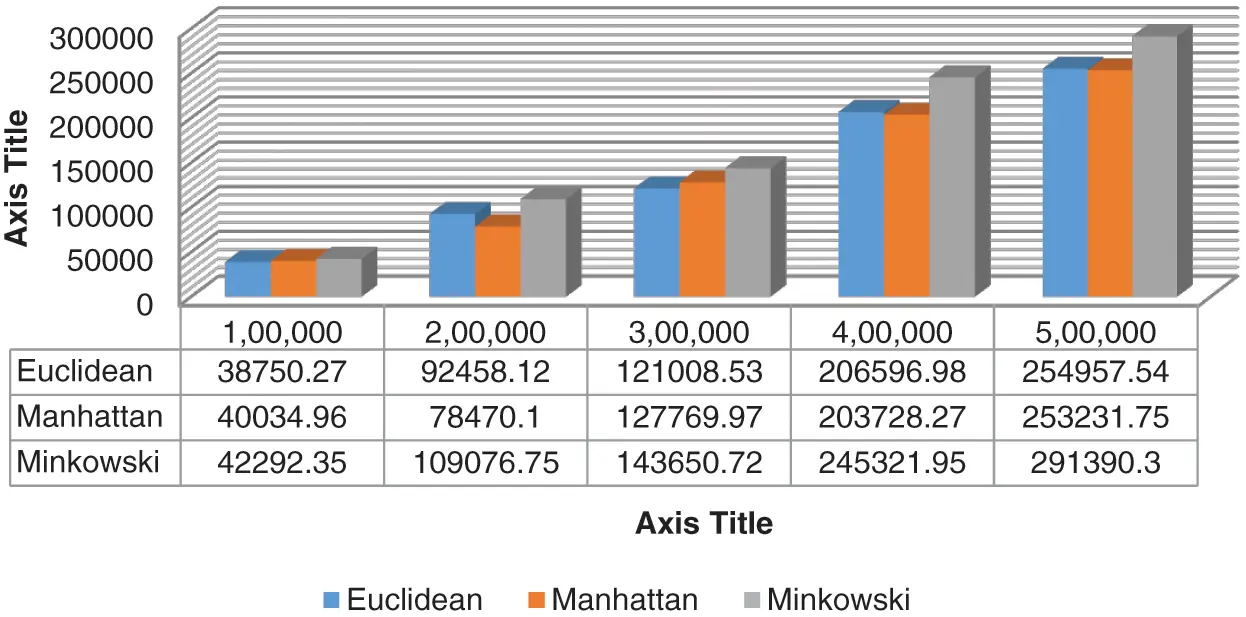Figure 1: Four Clusters, Running time of K-means algorithm with three different distance metrics

In Fig. 2, compared the running time of the K-means algorithm with three different distance metrics like Euclidean, Manhattan, and Minkowski. It is observed that Euclidean and Minkowski’s methods take the same time at 500,000 dataset points. But in fewer points, the Euclidean distance metric performs better results as compared to other metrics.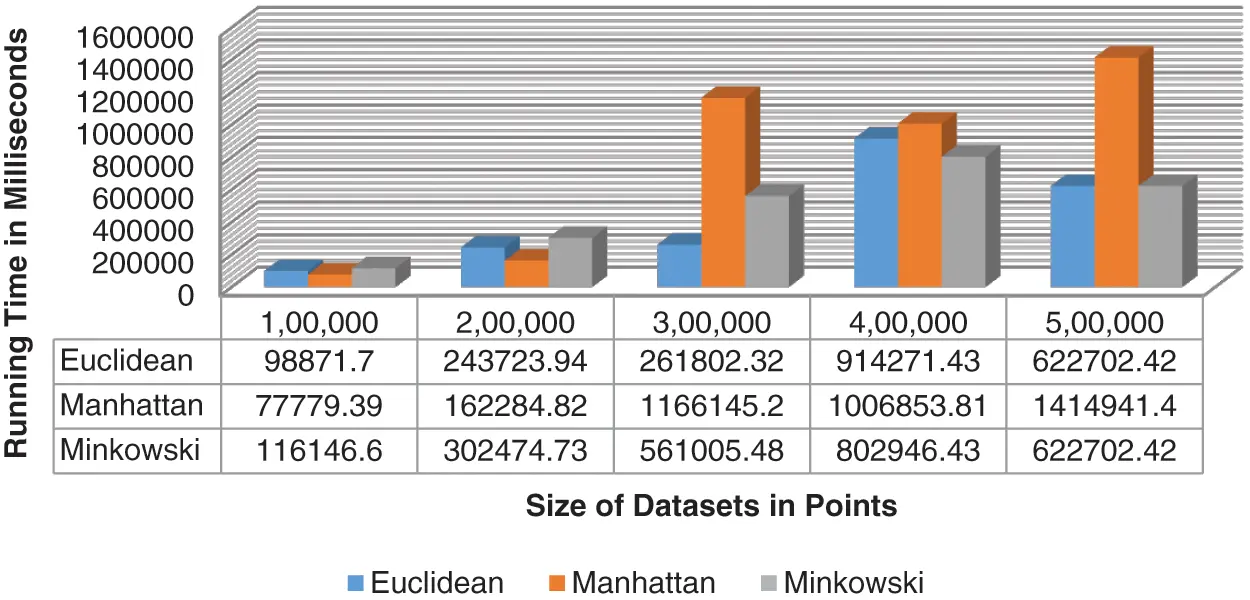Figure 2: Six Clusters, Running time of k-means algorithm with three different distance metrics

In Figs. 37, the running time is compared with three different distance metrics, where different sizes of datasets were splits into 8, 10, 12, 14, and 16 clusters, respectively. Running time is shown along the y-axis in milliseconds, and the compared different datasets of three different distance metrics are shown along the x-axis. It can be seen from experiments that Manhattan Distance performs better for 4, 8, 12, and 14 clusters. Euclidean Distance shows better for 6 and 16 clusters, while Minkowski Distance performs better only for 10 clusters.Figure 3: Eight Clusters, Running time of k-means algorithm with three different distance metrics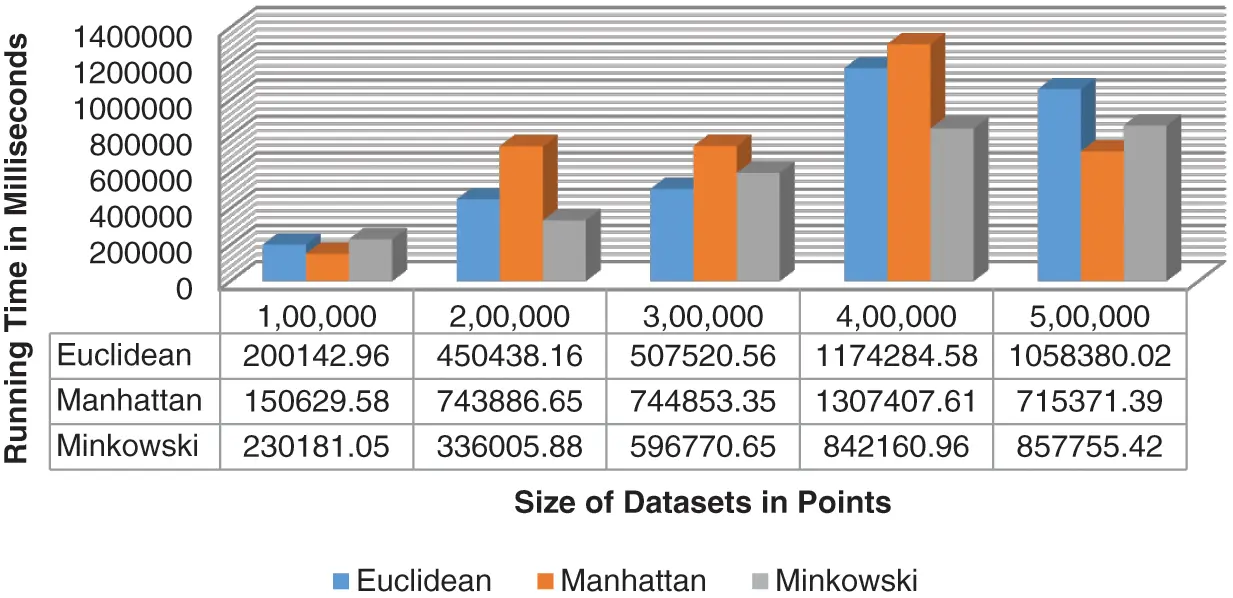Figure 4: Ten Clusters, Running time of k-means algorithm with three different distance metrics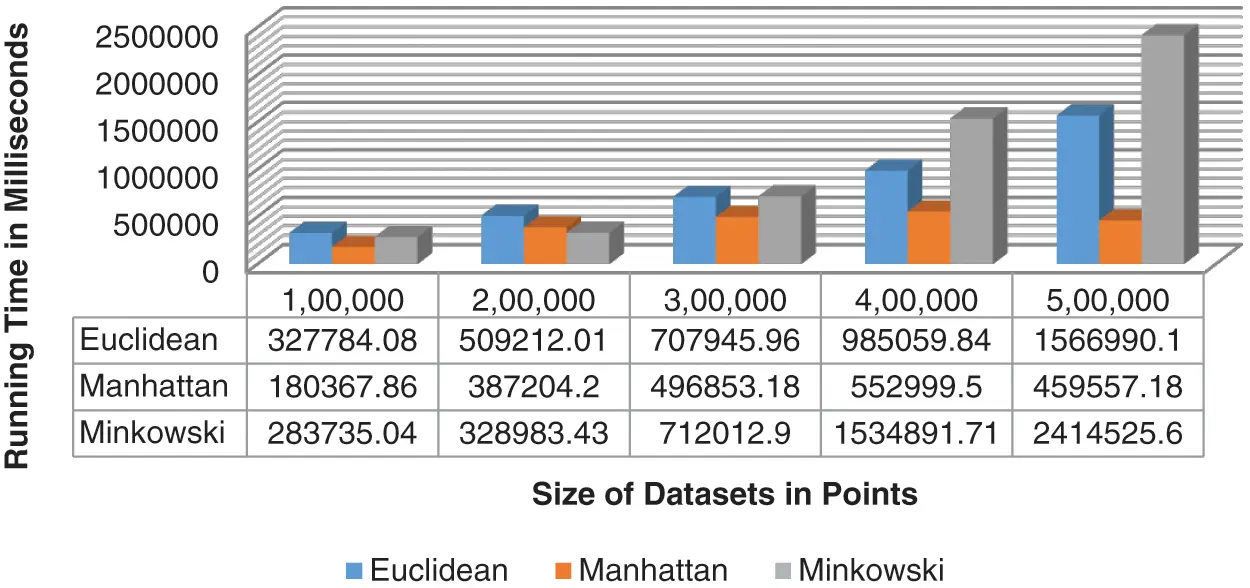Figure 5: Twelve Clusters, Running time of k-means algorithm with three different distance metrics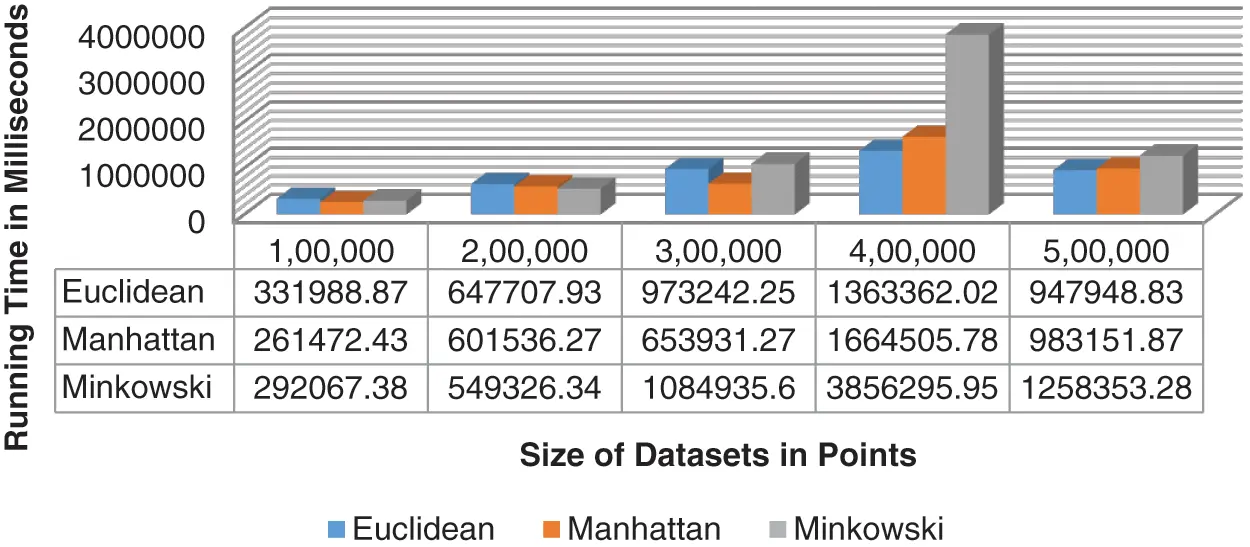Figure 6: Fourteen Clusters, Running time of k-means algorithm with three different mathematical models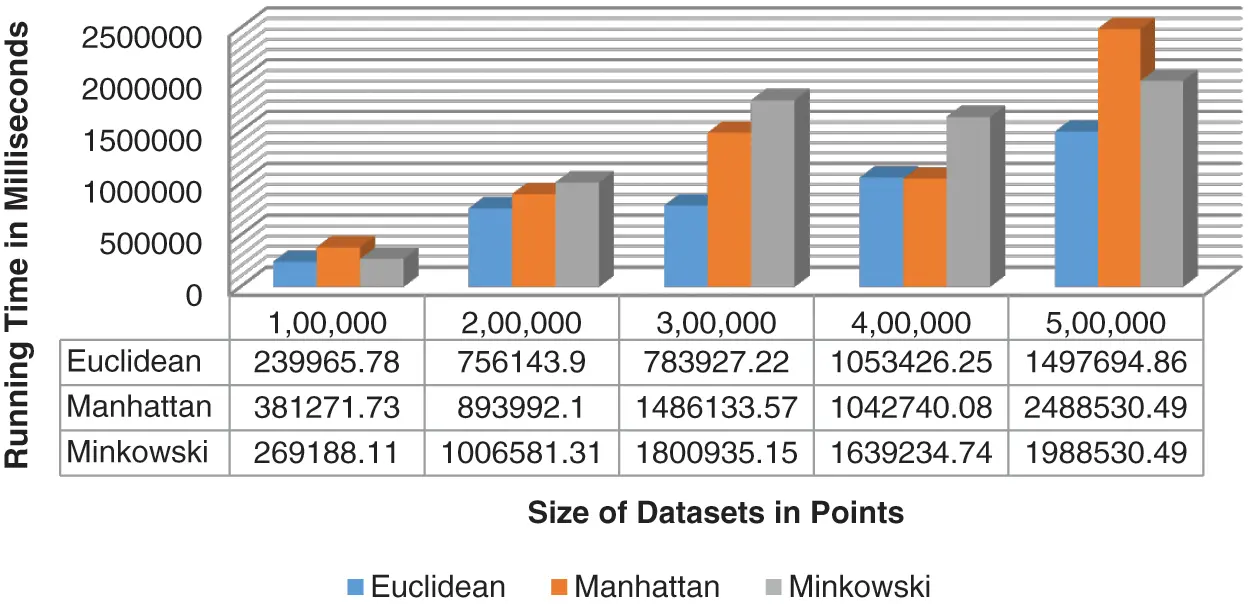Figure 7: Sixteen Clusters, Running time of k-means algorithm with three different distance metrics

6  Conclusions

One of the most popular clustering algorithms is K-means, where different distance metrics are used to find similar data objects. Distance is measured between every point of the dataset and centroids to assign the nearest cluster. In the experiments, the performances of three different metrics (Minkowski Distance, Manhattan Distance, and Euclidean Distance) were measured and compared in terms of execution time with different datasets and different numbers of clusters, i.e., 4, 6, 8, 10, 12, 14, and 16 clusters. It can be seen from experiments that Manhattan Distance performs better for 4, 8, 12, and 14 clusters. Euclidean Distance shows better for 6 and 16 clusters, while Minkowski Distance performs better only for 10 clusters. Overall Manhattan Distance performs better result. In future work, we will try to extend our approach to another partitioned-based clustering algorithm like K-Medoids, CLARA, and CLARANS.

Acknowledgement: Thanks to our families and colleagues, who provided moral support. We appreciate the linguistic assistance provided by TopEdit (www.topeditsci.com) during the preparation of this manuscript.

Funding Statement: The authors received no specific funding for this study.

Conflicts of Interest: The authors declare that they have no conflicts of interest to report regarding the present study.

## References

1. H. Rehioui, A. Idrissi, M. Abourezq and F. Zegrari, “Denclue-im: a new approach for big data clustering,” Procedia Computer Science, vol. 83, pp. 560–567, 2016.
2. B. Zerhari, A. A. Lahcen and S. Mouline, “Big data clustering: Algorithms and challenges,” in International Conference on Big Data, Cloud and Applications, Tetuan, Morocco, pp. 1–8, 2015.
3. T. Sajana, C. S. Rani and K. V. Narayana, “A survey on clustering techniques for big data mining,” Indian Journal of Science and Technology, vol. 9, no. 3, pp. 10–16, 2016.
4. M. Wu, X. Li, C. Liu, M. Liu, N. Zhao et al., “Robust global motion estimation for video security based on improved k-means clustering,” Journal of Ambient Intelligence and Humanized Computing, vol. 10, no. 2, pp. 439–448, 2019.
5. R. Jothi, S. K. Mohanty and A. Ojha, “Dk-means: a deterministic k-means clustering algorithm for gene expression analysis,” Pattern Analysis and Applications, vol. 22, no. 2, pp. 649–667, 2019.
6. T. Velmurugan and T. Santhanam, “A survey of partition-based clustering algorithms in data mining: an experimental approach,” Information Technology Journal, vol. 10, no. 3, pp. 478–484, 2011.
7. M. K. Arzoo and K. Rathod, “K-means algorithm with different distance metrics in spatial data mining with uses of netbeans ide 8. 2,” International Research Journal of Engineering and Technology, vol. 4, no. 4, pp. 2363–2368, 201
8. G. Tzortzis and A. Likas, “The min-max k-means clustering algorithm,” Pattern Recognition, vol. 47, no. 7, pp. 2505–2516, 2014.
9. F. U. Siddiqui and N. M. Isa, “Optimized k-means clustering algorithm for image segmentation,” Opto-Electronics Review, vol. 20, no. 3, pp. 216–225, 2012.
10. M. E. Celebi, H. A. Kingravi and P. A. Vela, “A comparative study of efficient initialization methods for the k-means clustering algorithm,” Expert Systems with Applications, vol. 40, no. 1, pp. 200–210, 2013.
11. H. Kaur and J. K. Sahiwal, “Image compression with an improved k-means algorithm for performance enhancement,” International Journal of Computer Science and Management Research, vol. 2, no. 6, pp. 1–8, 2016.
12. M. A. D. N. D. Harale and U. L. Kulkarni, “An iterative improved k-means clustering,” in International Conference on Advances in Computer Engineering, Kerala, India, pp. 25–28, 2011.
13. M. Sakthi and S. T. Antony, “An effective determination of initial centroids in k-means clustering using kernel PCA,” International Journal of Computer Science and Information Technologies, vol. 2, no. 3, pp. 955–959, 2011.
14. A. Fahad, N. Alshatri, Z. Tari, A. Alamri, I. Khalil et al., “A survey of clustering algorithms for big data: taxonomy and empirical analysis,” IEEE Transactions on Emerging Topics in Computing, vol. 2, no. 3, pp. 267–279, 20
15. M. Bora, D. Jyoti, D. Gupta and A. Kumar, “Effect of different distance measures on the performance of k-means algorithm: an experimental study in matlab,” ArXiv Preprint ArXiv, vol. 2014, pp. 1–9, 2014.
16. R. Loohach and K. Garg, “Effect of distance functions on k-means clustering algorithm,” International Journal of Computer Applications, vol. 50, no. 1, pp. 1–8, 2012.
17. P. Rathore and D. Shukla, “Analysis and performance improvement of k-means clustering in the big data environment,” in IEEE International Conference on Communication Networks, London, pp. 43–46, 2015.
18. S. Saqib, A. Ditta, M. A. Khan, S. A. R. Kazmi, H. Alquhayz et al., “Intelligent dynamic gesture recognition using cnn empowered by edit distance,” Computers Materials & Continua, vol. 66, no. 2, pp. 2061–2076, 2021.
19. B. S. Charulatha, P. Rodrigues, T. Chitralekha and A. Rajaraman, “A comparative study of different distance metrics that can be used in fuzzy clustering algorithms,” in National Conference on Architecture, Software Systems and Green Computing, Tamil Nadu, India, pp. 1–9, 2013.This work is licensed under a Creative Commons Attribution 4.0 International License, which permits unrestricted use, distribution, and reproduction in any medium, provided the original work is properly cited.